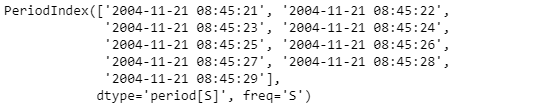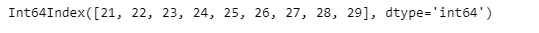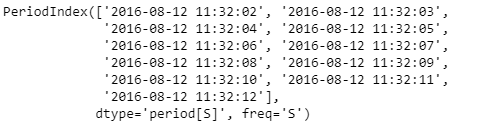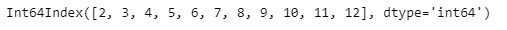# Python | Pandas PeriodIndex.second

Python is a great language for doing data analysis, primarily because of the fantastic ecosystem of data-centric python packages. Pandas is one of those packages and makes importing and analyzing data much easier.

Pandas` PeriodIndex.second` attribute return an Index object containing the second values for each period element present in the given PeriodIndex object.

Syntax : PeriodIndex.second

Parameters : None

Return : Index object

Example #1: Use `PeriodIndex.second` attribute to find out the second values present in each period element in the given PeriodIndex object.

 `# importing pandas as pd ` `import` `pandas as pd ` ` `  `# Create the PeriodIndex object ` `pidx ``=` `pd.PeriodIndex(start ``=``'2004-11-21 08:45:21 '``,  ` `               ``end ``=``'2004-11-21 8:45:29'``, freq ``=``'S'``) ` ` `  `# Print the PeriodIndex object ` `print``(pidx) `

Output :Now we will use the `PeriodIndex.second` attribute to find out the second values for each period element in pidx object.

 `# return the second values ` `pidx.second `

Output :As we can see in the output, the `PeriodIndex.second` attribute has returned seconds object containing the seconds value for each period in the given PeriodIndex object.

Example #2: Use `PeriodIndex.second` attribute to find out the second values present in each period element in the given PeriodIndex object.

 `# importing pandas as pd ` `import` `pandas as pd ` ` `  `# Create the PeriodIndex object ` `pidx ``=` `pd.PeriodIndex(start ``=``'2016-8-12 11:32:02'``,  ` `            ``end ``=``'2016-08-12 11:32:12'``, freq ``=``'S'``) ` ` `  `# Print the PeriodIndex object ` `print``(pidx) `

Output :Now we will use the `PeriodIndex.second` attribute to find out the second values for each period element in pidx object.

 `# return the second values ` `pidx.second `

Output :As we can see in the output, the `PeriodIndex.second` attribute has returned an Index object containing the seconds value for each period in the given PeriodIndex object.

My Personal Notes arrow_drop_upCheck out this Author's contributed articles.

If you like GeeksforGeeks and would like to contribute, you can also write an article using contribute.geeksforgeeks.org or mail your article to contribute@geeksforgeeks.org. See your article appearing on the GeeksforGeeks main page and help other Geeks.

Please Improve this article if you find anything incorrect by clicking on the "Improve Article" button below.

Article Tags :

Be the First to upvote.

Please write to us at contribute@geeksforgeeks.org to report any issue with the above content.